```EVR.include("level/summary/graph/Bar.js");
EVR.Level.Summary.Graph.Plot = function(graph, sets)
{
this.graph = graph;
this.sets = sets;
this.plot();
}
EVR.Level.Summary.Graph.Plot.prototype.plot = function()
{
var graph = this.graph;
var sets = this.sets;
var set, cell, bar, bars = [];
for (var ii = 0; ii < sets.measurements.length; ii++)
{
for (var jj = 0; jj < sets.length; jj++)
{
set = sets[jj];
measurement = set.measurements[ii];
cell = graph.insert_plot_cell(jj == sets.length - 1);
if (ii == 0 && jj == 0)
{
}
bar = new EVR.Level.Summary.Graph.Bar(this, cell, measurement, set);
cell.appendChild(bar.element);
bars.push(bar);
}
}
this.bars = bars;
}
EVR.Level.Summary.Graph.Plot.prototype.draw = function()
{
var bars = this.bars;
for (var ii = 0; ii < bars.length; ii++)
{
bars[ii].draw();
}
}
EVR.Level.Summary.Graph.Plot.prototype.toString = function()
{
return "[object EVR.Level.Summary.Graph.Plot]";
}
```
```EVR.Level.Summary.Graph.Bar = function(plot, cell, measurement, set)
{
this.plot = plot;
this.cell = cell;
this.measurement = measurement;
this.set = set;
this.element = document.createElement("div");
this.style();
this.set_cell_height();
this.set_height();
}
EVR.Level.Summary.Graph.Bar.prototype.style = function()
{
var element = this.element;
element.style.width = GRAPH_BAR_WIDTH;
element.style.background = this.set.color;
element.style.fontSize = "0px";
}
EVR.Level.Summary.Graph.Bar.prototype.set_cell_height = function()
{
var height = GRAPH_PLOT_HEIGHT * this.plot.graph.field.get_dimensions();
this.cell_height = height;
this.cell.style.height = height;
}
EVR.Level.Summary.Graph.Bar.prototype.set_height = function()
{
var set = this.set;
var worst = set.worst;
var range = set.range;
var difference = Math.abs(this.measurement - worst);
var max = this.cell_height - GRAPH_PLOT_MARGIN;
var min = GRAPH_MIN_BAR_HEIGHT;
if (range == 0)
{
var height = max;
}
else
{
var height = ((max - min) * (difference / range)) + min;
}
this.element.style.height = height + "px";
}
EVR.Level.Summary.Graph.Bar.prototype.draw = function()
{
this.set_cell_height();
this.set_height();
}
EVR.Level.Summary.Graph.Bar.prototype.toString = function()
{
return "[object EVR.Level.Summary.Graph.Bar]";
}
```
```EVR.Level.Summary.Comparison.Model = function(comparison, color, text)
{
this.field = comparison.field;
this.color = color;
this.text = text;
this.cell = comparison.row.insertCell(-1);
this.cell.style.textAlign = "center";
this.set_proportions();
}
{
var text = this.text;
var family = MODEL_FONT_FAMILY;
var color = MODEL_FONT_COLOR;
var size = MODEL_HEADING_FONT_SIZE;
var heading = new EVR.Text(this.cell, text, family, color, size, this.field);
var style = heading.element.style;
var border = MODEL_BORDER;
style.borderRight = border;
style.borderBottom = border;
style.borderLeft = border;
}
{
var model = document.createElement("div");
var style = model.style;
var border = MODEL_BORDER;
style.background = this.color;
style.borderTop = border;
style.borderRight = border;
style.borderLeft = border;
this.cell.appendChild(model);
this.model = model;
}
EVR.Level.Summary.Comparison.Model.prototype.set_proportions = function()
{
var height = this.field.get_dimensions();
var model_height = height * MODEL_HEIGHT;
var style = this.model.style;
style.height = model_height;
style.width = model_height;
this.cell.style.width = model_height;
}
EVR.Level.Summary.Comparison.Model.prototype.draw = function()
{
this.set_proportions();
}
EVR.Level.Summary.Comparison.Model.prototype.toString = function()
{
return "[object EVR.Level.Summary.Comparison.Model]";
}
```
```EVR.include("level/summary/comparison/Model.js");
EVR.Level.Summary.Comparison = function(row, level)
{
this.row = row;
this.field = level.container;
}
{
var row = this.row;
var painter = this.painter;
var actual = painter.blend().get_string();
var expected = painter.blend(1).get_string();
var actual = new EVR.Level.Summary.Comparison.Model(
this, actual, MODEL_ACTUAL_TEXT);
var expected = new EVR.Level.Summary.Comparison.Model(
this, expected, MODEL_EXPECTED_TEXT);
this.models = [actual, expected];
}
EVR.Level.Summary.Comparison.prototype.draw = function()
{
var models = this.models;
for (var ii = 0; ii < models.length; ii++)
{
models[ii].draw();
}
}
EVR.Level.Summary.Comparison.prototype.toString = function()
{
return "[object EVR.Level.Summary.Comparison]";
}
```
```EVR.Level.Scoreboard.Speed = function()
{
this.sum = 0;
this.measurements = 0;
this.average = null;
}
{
this.sum += parseFloat(speed);
this.measurements++;
}
EVR.Level.Scoreboard.Speed.prototype.updateAverage = function()
{
this.average = this.sum / this.measurements;
}
EVR.Level.Scoreboard.Speed.prototype.getAverage = function()
{
return this.average;
}
```
18.207.252.123
18.207.252.123
18.207.252.123

September 30, 2015

Edge of Life is a form I made with Babycastles and Mouth Arcade for an event in New York called Internet Yami-ichi, a flea market of internet-ish goods. We set up our table to look like a doctor's office and pharmacy and offered free examinations and medication prescriptions, a system described by one person as "a whole pharmacy and medical industrial complex".

Diagnoses were based on responses to the form and observations by our doctor during a short examination. The examination typically involved bizarre questions, toy torpedoes being thrown at people and a plastic bucket over the patient's head. The form combined ideas from Myers-Briggs Type Indicators, Codex Seraphinianus and chain-mail personality tests that tell you which TV show character you are. In our waiting room, we had Lake of Roaches installed in a stuffed bat (GIRP bat). It was really fun!

The icons for the food pyramid are from Maple Story and the gun icons are from the dingbat font Outgunned. I'm also using Outgunned to generate the items in Food Spring.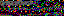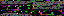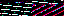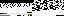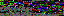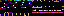↠ RSS Feed ↞